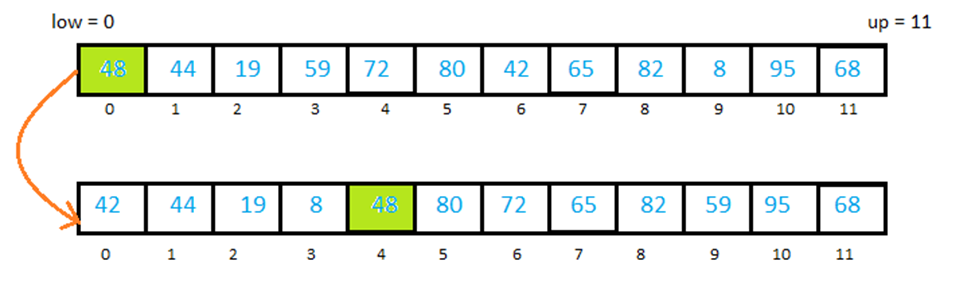Here you get sort program and algorithm.

Quick sort is based on divide and Conquer technique. We divide our array into sub-arrays and that sub-arrays divided into another sub-arrays and so on, until we get smaller arrays. Because it is easy to arrays in compare to a large array.

Sorting smaller arrays will sort the entire array.

Python Quick Sort

To understand Quick Sort let’s  take an example:-

Example

We have an array [48,44,19,59,72,80,42,65,82,8,95,68]

First of all we take first element and place it at its proper place. We call this element Pivot element.

Note: We can take any element as Pivot element but for convenience the first element is taken as Pivot.

There are two condition to place Pivot at its proper place.

• All the elements to the left of Pivot element should be smaller than
• All the elements to the right of Pivot element should be greater than

In given array, we’ll take first element as Pivot element which is 48. Now place it at its proper place according to first two conditions.Here all the elements to the left is smaller and to right are greater than Pivot.

If you confused that how we placed Pivot at its proper place then wait we’ll discuss it later in partition algorithm.

Well, now we’ll take two sub-lists/sub-arrays left and right of our Pivot element (48).  Sub-lists will be

[42,44,19,8] and [80,72,65,82,59,95,68]

and will also take first element as Pivot element in both and place the their Pivot elements at their proper places using partition algorithm. After this step each of the sub-lists will be divided into two parts then new sub-lists will be divided into another two parts and so on until we reached the end.

Let’s see how it will look like.Pivot element represented by Green color.

We can write a recursive function for this procedure. There would be two terminating conditions. When the sub-list has only 1 element or when no sub-list is formed. If the value of low is equal to up then there will be only one element in the sub-list and if the value of low exceeds up then no sub-list is formed. So our algorithm will be.

Algorithm

Quick[array, low,up]

Where low and up are lower and upper bound of the array.

STEP 1:    if low>=up then return

STEP 2:    set piv_loc = call partition(array,low,up)

[calling partition to find the of pivot]

STEP 3:    call Quick(array,low,piv_loc-1)

[Calling Quick for left sub-list]

STEP 4:    call Quick(array,piv_loc+1, up)

[Calling Quick for right sub-list]

Partition [array, low, up]

This algorithm is to find the location of pivot element and return it.

STEP 1:  set i = low+1

Set j = up

Set pivot = array[low]

STEP 2:  while(i <= j)

{

While(( array[i] < pivot ) and ( i < up ))

Increase i by 1

While ( array[j] > pivot )

Decrease j by 1

If ( i < j )

{

Swap the value of array[i] with array[j]

Increase i by 1

Decrease j by 1

}

Else

Increase i by 1

}

STEP 3:    set array[low] = array[j]

Set array[j] = pivot

Return j

Time Complexity

Best Case: O (n log2n)

Average Case: O (n log n)

Worst Case: O (n2)

Program for Quick Sort in Python

Output

8 19 42 44 48 59 65 68 72 80 82 95

Comment below if you have doubts related to above python quicksort tutorial.

SHARE
Previous articleMathematical Genealogy | Math ∩ Programming